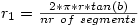Original math for Polar Grids

The angle of the polar grid is kept constant with the following theory.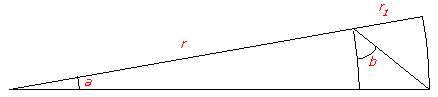When a is small and r large, the small edge of the pie could be considered straight.

With b = 45 degrees, r1 equals the small pie edge.

The approximation above in math: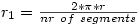For the circle we get: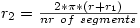From the mathematical rule: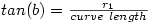we can deduce: